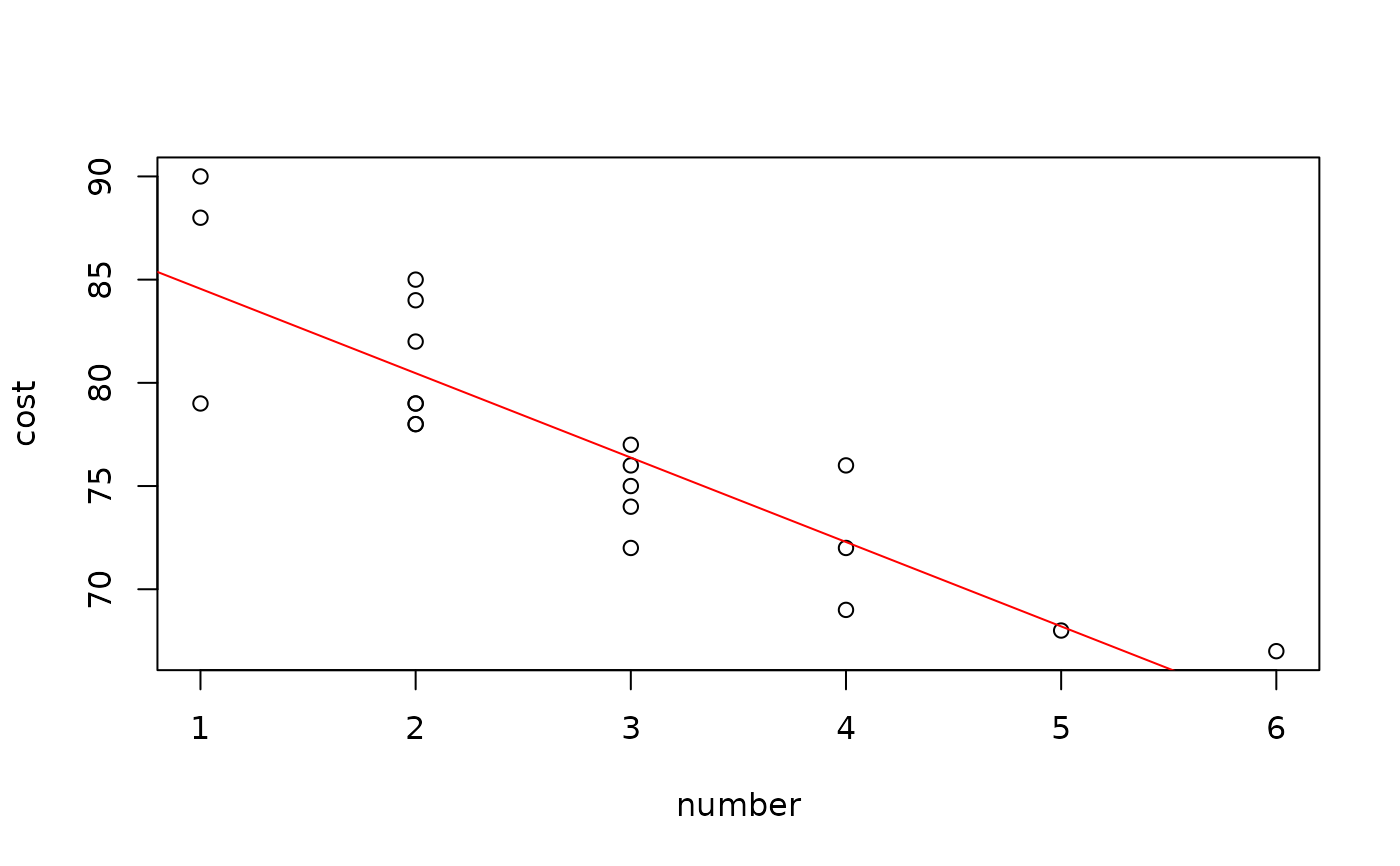Data for Exercise 2.89

Family

## Format

A data frame/tibble with 20 observations on two variables

number

number in family

cost

cost per person (in dollars)

## Examples


plot(cost ~ number, data = Family)
abline(lm(cost ~ number, data = Family), col = "red")cor(Family$cost, Family$number)
#>  -0.8680791

if (FALSE) {
library(ggplot2)
ggplot2::ggplot(data = Family, aes(x = number, y = cost)) +
geom_point() +
geom_smooth(method = "lm") +
theme_bw()
}